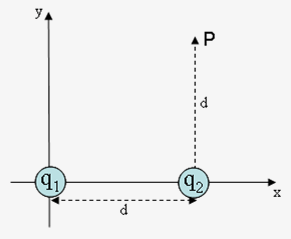# Problem: Two point charges (q1 = -4.7 µC and q2 = 8.4 µC) are fixed along the x-axis, separated by a distance d = 8.1 cm. Point P is located at (x,y) = (d,d).1) What is Ex(P), the value of the x-component of the electric field produced by q1 and q2 at point P?2) What is Ey(P), the value of the y-component of the electric field produced by q1 and q2 at point P?

###### FREE Expert Solution

Electric field for a point charge:

$\overline{){\mathbf{E}}{\mathbf{=}}\frac{\mathbf{k}\mathbf{q}}{{\mathbf{r}}^{\mathbf{2}}}\stackrel{\mathbf{^}}{\mathbf{r}}}$

1)

For multiple charges:

Enet x = E1x + E2x = E1cosθ1 + E2cosθ2

91% (321 ratings)###### Problem Details

Two point charges (q1 = -4.7 µC and q2 = 8.4 µC) are fixed along the x-axis, separated by a distance d = 8.1 cm. Point P is located at (x,y) = (d,d).1) What is Ex(P), the value of the x-component of the electric field produced by q1 and q2 at point P?

2) What is Ey(P), the value of the y-component of the electric field produced by q1 and q2 at point P?